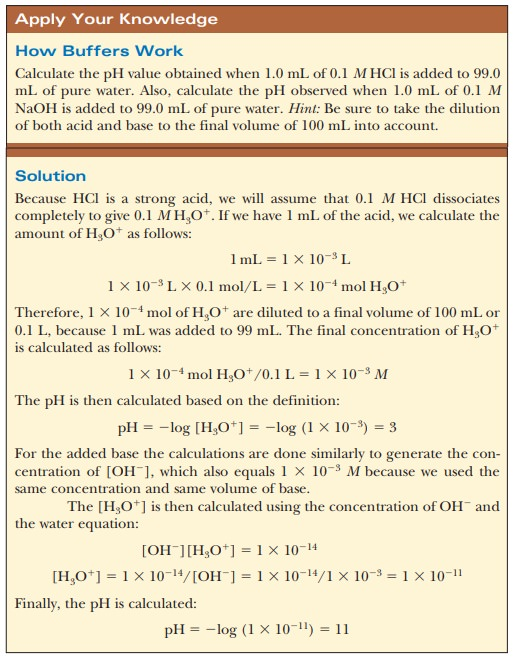Home | | Biochemistry | How do buffers work?

# How do buffers work?Let us compare the changes in pH that occur on the addition of equal amounts of strong acid or strong base to pure water at pH 7 and to a buffer solution at pH 7.

## How do buffers work?

Let us compare the changes in pH that occur on the addition of equal amounts of strong acid or strong base to pure water at pH 7 and to a buffer solution at pH 7. If 1.0 mL of 0.1 M HCl is added to 99.0 mL of pure water, the pH drops drastically. If the same experiment is conducted with 0.1 M NaOH instead of 0.1 HCl, the pH rises drastically (Figure 2.14).The results are different when 99.0 mL of buffer solution is used instead of pure water. A solution that contains the monohydrogen phosphate and dihydrogen phosphate ions, HPO42- and H2PO4-, in suitable proportions can serve as such a buffer. The Henderson–Hasselbalch equation can be used to calculate the HPO42-/H2PO4- ratio that corresponds to pH 7.0.For purposes of illustration, let us consider a solution in which the concen-trations are [HPO42-= 0.063 M and [H2PO4-= 0.10 M;this gives the conjugate base/weak acid ratio of 0.63 seen previously. If 1.0 mL of 0.10 M HCl is added to 99.0 mL of the buffer, the reaction

[HPO42-1 H+ ßà H2PO4-

takes place, and almost all the added H+ will be used up. The concentrations of [HPO42-] and [H2PO4-] will change, and the new concentrations can be calculated.The new pH can then be calculated using the Henderson-Hasselbalch equation and the phosphate ion concentrations. The appropriate pKais 7.20 (Table 2.6).The new pH is 6.99, a much smaller change than in the unbuffered pure water (Figure 2.14). Similarly, if 1.0 mL of 0.1 M NaOH is used, the same reaction takes place as in a titration:Almost all the added OH- is used up, but a small amount remains. Because this buffer is an aqueous solution, it is still true that Kw=[H+][OH-]. The increase in hydroxide ion concentration implies that the hydrogen ion concentration decreases and that the pH increases. Use the Henderson–Hasselbalch equation to calculate the new pH and to convince yourself that the result is pH 5 7.01, again a much smaller change in pH than took place in pure water (Figure 2.14). Many biological reactions will not take place unless the pH remains within fairly narrow limits, and, as a result, buffers have great practical importance in the biochemistry laboratory.

Study Material, Lecturing Notes, Assignment, Reference, Wiki description explanation, brief detail
Biochemistry: Water: The Solvent for Biochemical Reactions : How do buffers work? |